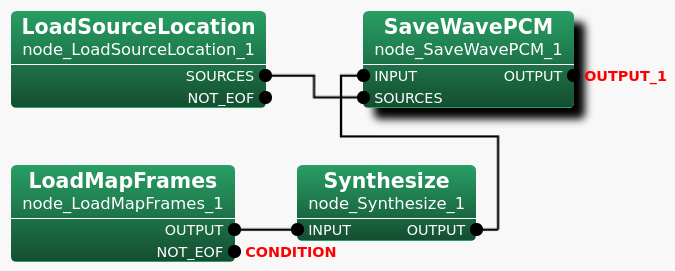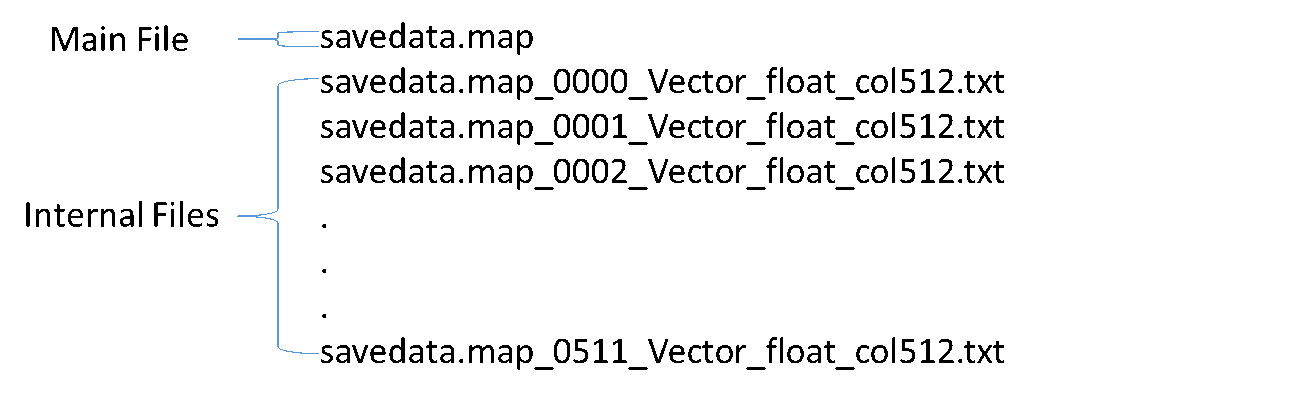6.7.5.1 Outline of the node

Loads data from files created by SaveMapFrames into containers in either the Map $<$int , Vector<float> $>$ type or the Map $<$int , Vector<complex<float> > $>$ type.

6.7.5.2 Necessary files

Data files in either text format (TEXT) or binary format (RAW) which are called "Internal files" and a text file which is called "Main file" containing lists of those data files per frame. All files were created by SaveMapFrames .

6.7.5.3 Usage

When to use

This nodes is used to use data of frames saved in files in advance by SaveMapFrames to process further such as converting the data into a wav file later.

Typical connection

Figure 6.109 below shows a connection example using LoadMapFrames in a network. The files that LoadMapFrames loads are to be specified by value in the FILENAME parameter. In this network, Synthesize takes data in the Map $<$int , Vector<complex<float> > $>$ type, which are output of LoadMapFrames , to convert them into waveforms of time domain. Then, SaveWavePCM saves the data outputted by Synthesize as a sound file. LoadSourceLocation is an optional in this network. The network gives an example that SaveWavePCM optionally takes sound source localization results in the Vector<ObjectRef> type as well. Note that the ObjectRef must refer to data in the Source type. LoadSourceLocation loads data from a file generated by SaveSourceLocation while LoadMapFrames loads data from files generated by SaveMapFrames .Figure 6.109: Connection example of LoadMapFrames

6.7.5.4 Input-output and property of the node

Input

No inputs.

Output

OUTPUT

: Map $<$int , Vector<float> $>$ or Map $<$int , Vector<complex<float> > $>$ of the Map<int, ObjectRef> type. Data of frames where the data are mapped to keys of the Map.

NOT_EOF

: bool type. A flag to indicate whether or not the end of file has been reached when a program is reading the "Main file" which contains lists of "Internal files" for each frame. It is set to false when the program reaches the end of file of the "Main file". Then, the program will be terminated. Otherwise, it is being set to true.

Parameter

Table 6.92: Parameter list of LoadMapFrames
 Parameter name Type Default value Unit Description FILENAME The name of the text file containing lists of data files for each frame. INPUT_TYPE TEXT_float The file format and the data type of the input data files. Select TEXT_float, RAW_float, TEXT_complex_float, or RAW_complex_float. COL_SIZE 512 The column size of the Vector of the Map $<$int , Vector $>$ type in which data in the data file are to be restored.
FILENAME

: string type. The name of the "Main file" containing lists of data files for each frame, created by SaveMapFrames . For more details on files generated by SaveMapFrames , see the node reference.

INPUT_TYPE

: string type. The file format and the data type of input data files. Select appropriate one from the drop-down menu. Four options are available as follows.

• TEXT_float

• RAW_float

• TEXT_complex_float

• RAW_complex_float

The default value is set to TEXT_float. If the wrong value is selected, the Network will not run successfully. Figure 6.110 gives sample file names for both "Main file" and "Internal files". Each file name of "Internal Files" indicates data type, "Vector_float" for this example. The file extension indicates the file format. Each file has its extension, either "txt" for text (TEXT) or "raw" for binary (RAW).

COL_SIZE

: int type. The Vector size of data in the Map $<$int , Vector<ObjectRef> $>$ type saved in the input data files which can be found in the name of the file to load as shown in Figure 6.110. If the wrong value is specified, the Network will not run successfully. The default value is 512.Figure 6.110: SaveMapFrames Generated Files

See the node reference of SaveMapFrames for more details on files generated by SaveMapFrames .

6.7.5.5 Details of the node

LoadMapFrames reads files generated by SaveMapFrames to restore the data in containers in either the Map $<$int , Vector<float> $>$ type or the Map $<$int , Vector<complex<float> > $>$ type. LoadMapFrames loads two types of files. One is data files which are called "Internal file" containing data of frames separated for each key. The other one is a text file which is called "Main file" contains lists of "Internal files" following by each frame number. LoadMapFrames obtains information from both the frame number and the filenames in "Main file" in order to restore data saved in "Internal file" properly into containers in the corresponding data type. Table 6.93 below shows the all available INPUT_TYPE parameter values and the corresponding output data types.

 INPUT_TYPE Output Type Text Float Map $<$int , Vector $>$ Raw Float Map $<$int , Vector $>$ Text Complex Float Raw Complex Float